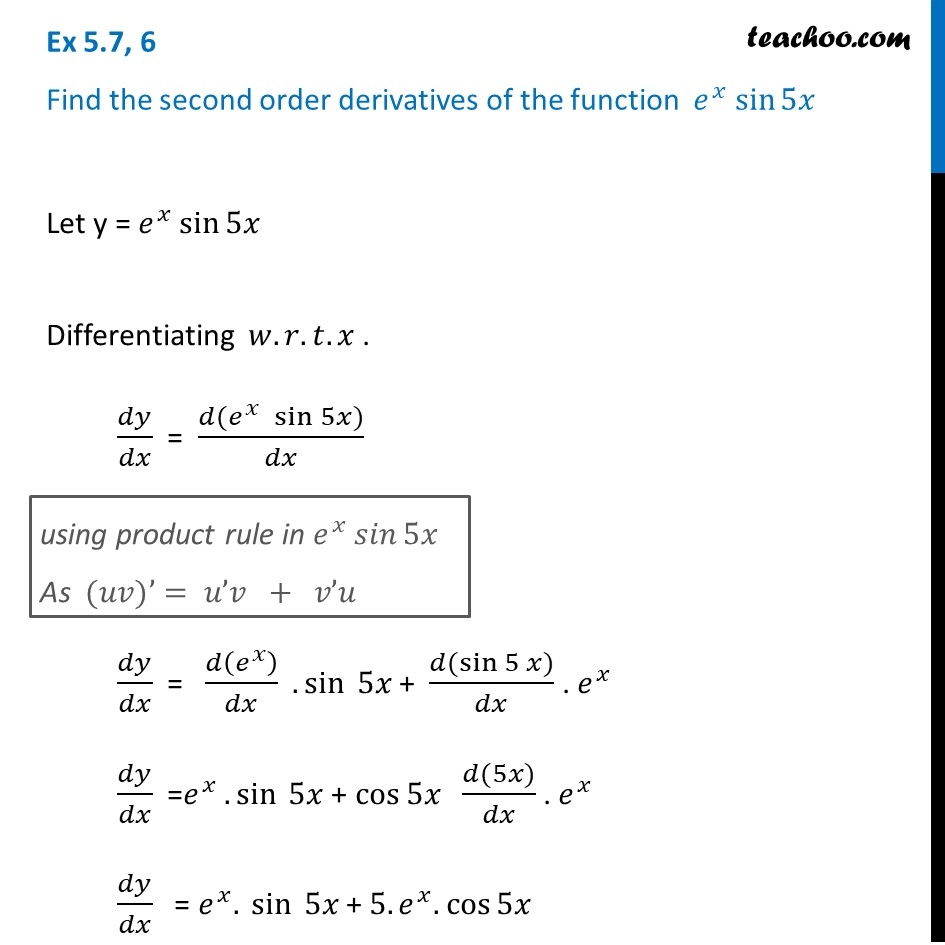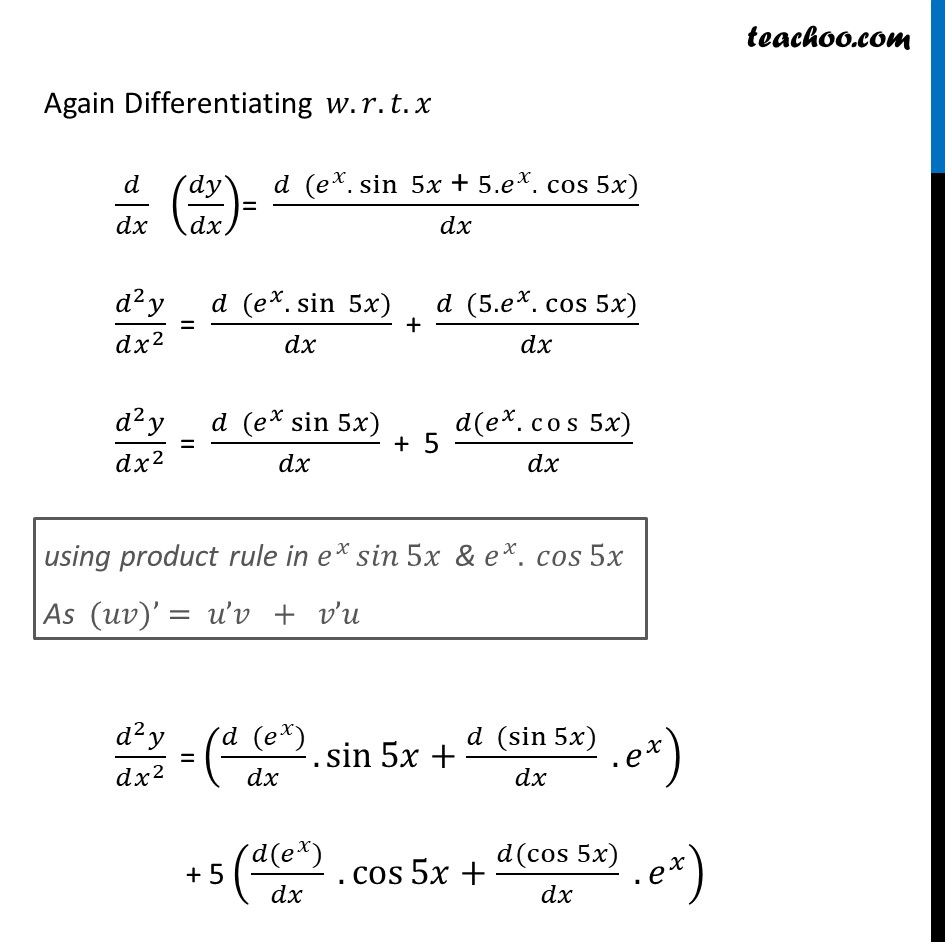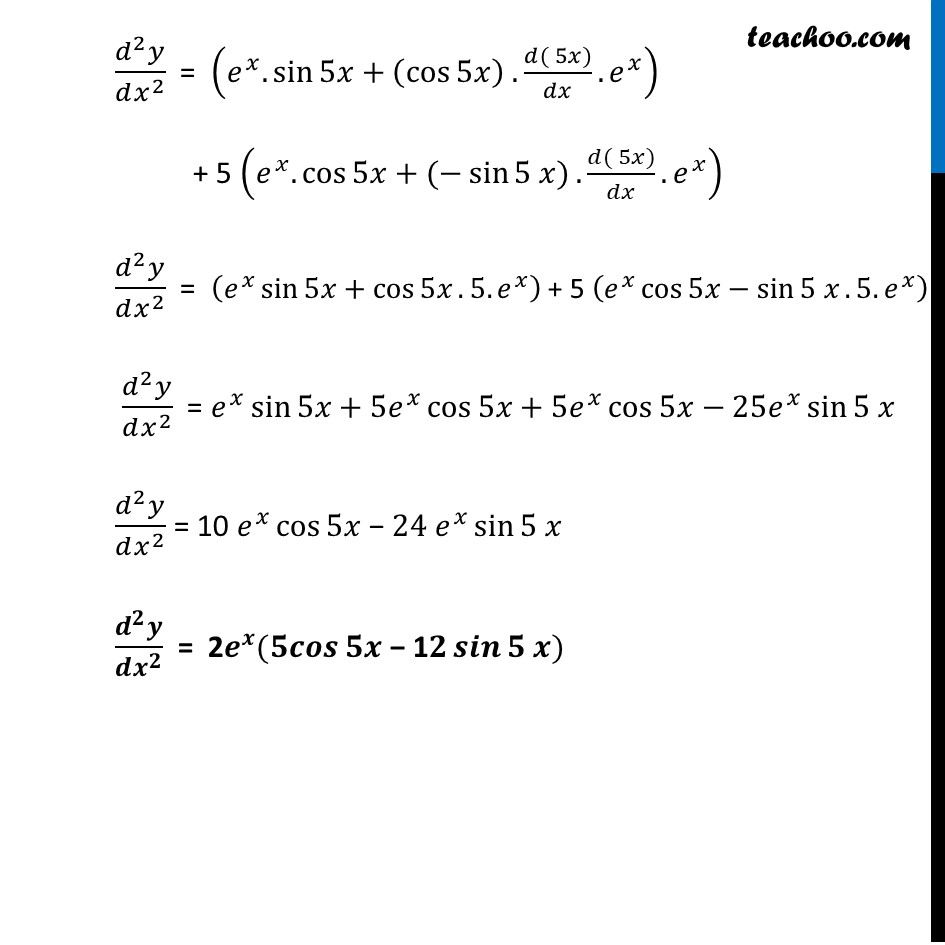Ex 5.7

Chapter 5 Class 12 Continuity and Differentiability
Serial order wiseLearn in your speed, with individual attention - Teachoo Maths 1-on-1 Class

### Transcript

Ex 5.7, 6 Find the second order derivatives of the function 𝑒^𝑥 sin⁡5𝑥 Let y = 𝑒^𝑥 sin⁡5𝑥 Differentiating 𝑤.𝑟.𝑡.𝑥 . 𝑑𝑦/𝑑𝑥 = (𝑑(𝑒^𝑥 " " sin⁡5𝑥))/𝑑𝑥 𝑑𝑦/𝑑𝑥 = 𝑑(𝑒^𝑥 )/𝑑𝑥 .sin⁡〖 5𝑥〗 + (𝑑(〖sin 5〗⁡𝑥))/𝑑𝑥 . 𝑒^𝑥 𝑑𝑦/𝑑𝑥 =𝑒^𝑥 .sin⁡〖 5𝑥〗 + cos⁡5𝑥 (𝑑(5𝑥))/𝑑𝑥 . 𝑒^𝑥 𝑑𝑦/𝑑𝑥 = 𝑒^𝑥. sin⁡〖 5𝑥〗 + 5.𝑒^𝑥. cos⁡5𝑥 using product rule in 𝑒^𝑥 𝑠𝑖𝑛⁡5𝑥 As (𝑢𝑣)’= 𝑢’𝑣 + 𝑣’𝑢 Again Differentiating 𝑤.𝑟.𝑡.𝑥 𝑑/𝑑𝑥 (𝑑𝑦/𝑑𝑥)= (𝑑 (𝑒^𝑥. sin⁡〖 5𝑥〗 " + " 5.𝑒^𝑥." " cos⁡5𝑥))/𝑑𝑥 (𝑑^2 𝑦)/(𝑑𝑥^2 ) = (𝑑 (𝑒^𝑥. sin⁡〖 5𝑥〗))/𝑑𝑥 + (𝑑 (5.𝑒^𝑥." " cos⁡5𝑥))/𝑑𝑥 (𝑑^2 𝑦)/(𝑑𝑥^2 ) = (𝑑 (𝑒^𝑥 sin⁡5𝑥))/𝑑𝑥 + 5 (𝑑(𝑒^𝑥." " cos⁡5𝑥))/𝑑𝑥 (𝑑^2 𝑦)/(𝑑𝑥^2 ) = ((𝑑 (𝑒^𝑥))/𝑑𝑥.sin⁡5𝑥+(𝑑 (sin⁡5𝑥))/𝑑𝑥 .𝑒^𝑥 ) + 5 ((𝑑(𝑒^𝑥))/𝑑𝑥 .cos⁡5𝑥+(𝑑(cos⁡5𝑥))/𝑑𝑥 .𝑒^𝑥 ) using product rule in 𝑒^𝑥 𝑠𝑖𝑛⁡5𝑥 & 𝑒^𝑥." " 𝑐𝑜𝑠⁡5𝑥 As (𝑢𝑣)’= 𝑢’𝑣 + 𝑣’𝑢 (𝑑^2 𝑦)/(𝑑𝑥^2 ) = (𝑒^𝑥.sin⁡5𝑥+(cos⁡5𝑥 ) .(𝑑(⁡5𝑥))/𝑑𝑥.𝑒^𝑥 ) + 5 (𝑒^𝑥.cos⁡5𝑥+(−sin⁡〖5 𝑥)〗.(𝑑(⁡5𝑥))/𝑑𝑥.𝑒^𝑥 ) (𝑑^2 𝑦)/(𝑑𝑥^2 ) = (𝑒^𝑥 sin⁡5𝑥+cos⁡5𝑥.5.𝑒^𝑥 ) + 5 (𝑒^𝑥 cos⁡5𝑥−sin⁡〖5 𝑥〗.5.𝑒^𝑥 ) (𝑑^2 𝑦)/(𝑑𝑥^2 ) = 𝑒^𝑥 sin⁡5𝑥+5𝑒^𝑥 cos⁡5𝑥+5𝑒^𝑥 cos⁡5𝑥−25𝑒^𝑥 sin⁡〖5 𝑥〗 (𝑑^2 𝑦)/(𝑑𝑥^2 ) = 10 𝑒^𝑥 cos⁡5𝑥 − 24 𝑒^𝑥 sin⁡〖5 𝑥〗 (𝒅^𝟐 𝒚)/(𝒅𝒙^𝟐 ) = 2𝒆^𝒙 (𝟓𝒄𝒐𝒔⁡𝟓𝒙 − 1𝟐 𝒔𝒊𝒏⁡〖𝟓 𝒙〗)